# Texas Go Math Grade 1 Unit 3 Answer Key Algebraic Reasoning

Refer to our Texas Go Math Grade 1 Answer Key Pdf to score good marks in the exams. Test yourself by practicing the problems from Texas Go Math Grade 1 Unit 3 Answer Key Algebraic Reasoning.

## Texas Go Math Grade 1 Unit 3 Answer Key Algebraic Reasoning

Show What You Know

Model More, Model Fewer

Question 1.
Circle the row that has more.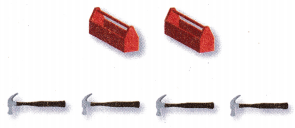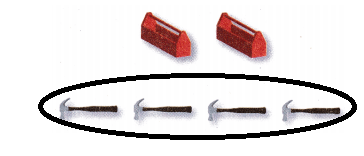Explanation:
2 boxes < 4 hammers

Question 2.
Circle the row that has fewer.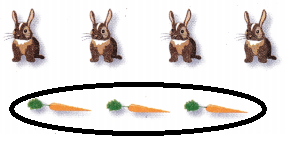Explanation:
4 rabits > 3 carrots

Count On

Use the number line to add. Write each sum.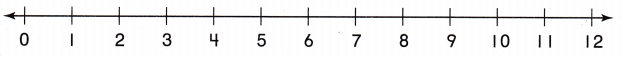Question 3.
6 + 3 = ____Explanation:
The Sum of 6 and 3 is 9
6 + 3 = 9

Question 4.
7 + 1 = ____Explanation:
The sum of 7 and 1 is 8
7 + 1 = 8

Question 5.
8 + 2 = ____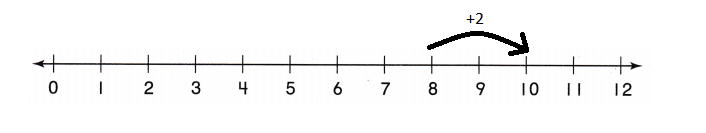Explanation:
The sum of 8 and 2 is 10
8 + 2 = 10

Count Back

Use the number line to subtract. Write each difference.Question 6.
11 – 2 = ____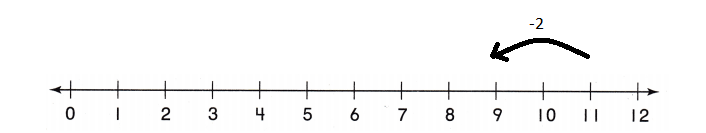Explanation:
The difference of 11 and 2 is 9
11 – 2 = 9

Question 7.
8 – 3 = ____Explanation:
The difference of 8 and 3 is 5
8 – 3 = 5

Question 8.
9 – 1 = ____Explanation:
The difference of 9 and 1 is 8
9 – 1 = 8

Vocabulary Builder

Visualize it

Sort the review words from the box.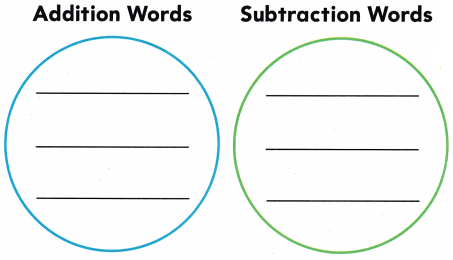Explanation:The words are organized according to the review words

Understand Vocabulary

Use a review word to complete each sentence.

Question 1.
8 is the _____________ for 17 – 9
Explanation:
8 is the subtract for 17 – 9
Question 2.
17 is the ____________ for 8 + 9.
Explanation:
17 is the addition for 8 + 9.

Question 3.
When you ____________ 4 to 8, you find the sum.
Explanation:
When you add 4 to 8, you find the sum as
4 + 8 = 12

Question 4.
5 ducks and 5 swans are at the pond. There are the ___________ number of ducks and swans.
Explanation:
5 ducks and 5 swans are at the pond. There are the 10 number of ducks and swans.
5 + 5=10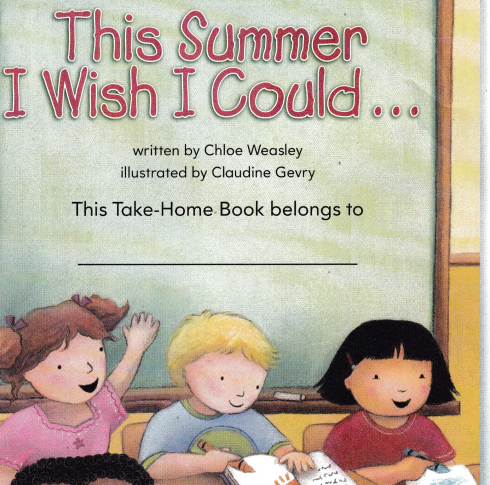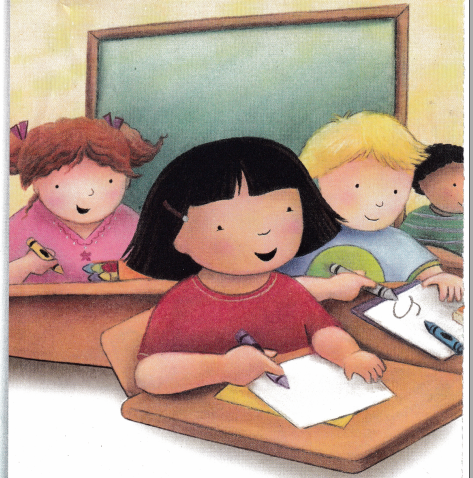To my surprise and great delight
Our teacher asked us to draw or write
About something we would think so cool
To do this summer when out of school.
So many ideas swirled ¡n my head!
“There are lots of things I’d do,” I said.Fd sail the ocean in a boat.
My first mate would be a goat.
From the starboard side we’d see
A pod of whales. There would be 3.
Then I’d spy 4 whales more!
Oh, such a summer I’d adore.

How many whales do they see in all?
_____ + _____ = _____ whales
Explanation:
3 + 4 = 7 whales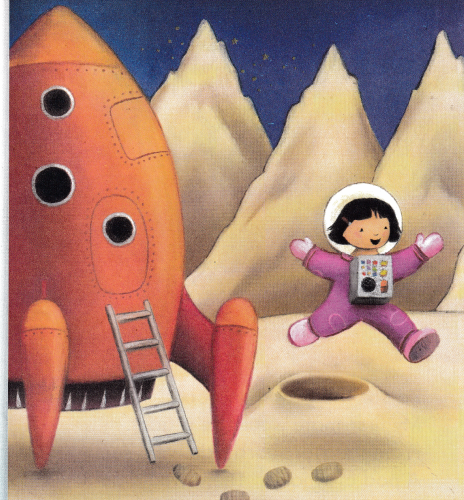I’d take o rocket to where it’s starry.
Oh, what fun, an outer space safari!
The first of many stops would be
Our moon, where moon mountains
I would see.How many moon mountains are tall?
1 mountain is tall
How many are short?
Explanation:
4 mountains are short
How many moon mountains are there in all?
____ + ____ = ____ moon mountains
1 + 4 = 5 moon mountainsOr perhaps this summer time,
My goat and I would take a climb.
Then build a house up in a tree.
A farm and far off city we could see.
How many cows? How many horses?
6 cows
and 2 horses
How many fewer horses than cows?
___ – ___ = ___ fewer horses
6 – 2 = 4 fewer horses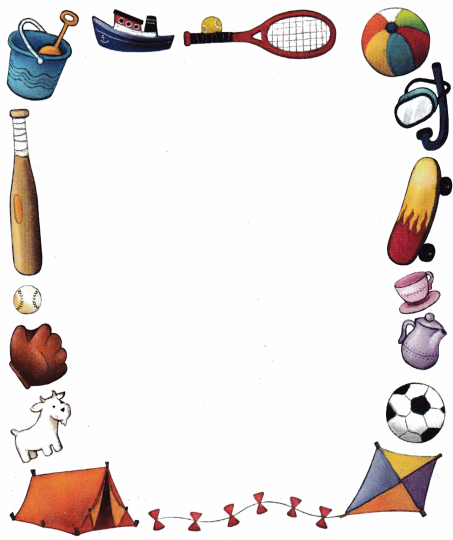Now it’s your turn to write or draw.Think of summer and what you’d do.
In summer we like to visit the mountains, valleys and snow fall, beach.
If you could plan what’s best for you!Ex
Explanation:7 – 4 = 3
so , three are pink whales.

More and Fewer

Look at the picture. Complete the number sentences.Question 1.
How many cows and horses are there?
___ + ___ = ____
4 + 2 = 6
Explanation.
6 cows and horses are there

Question 2.
How many more cows are there than horses?
____ – ____ = ____ more cows
6 – 2 = 4 more cows
Explanation:
4 more cows are there than horses

Question 3.
Four cows go into the barn. How many cows are there now?
___ – ____ =____ cows
4 – 4 = 0
0 cows
Explanation:
0 cows are there now

Math Board Write a story problem about animals you see on a farm. Use the words more or fewer in your story. Have a classmate solve the problem.
Explanation:
There are 6 animals in the farm.
4 are cows and 2 are horses
How many fewer horses are there than cows.